Question

# Why do new classical economists argue that in the long run aggregate demand can only affect...

Why do new classical economists argue that in the long run aggregate demand can only affect the price level? Use a diagram to illustrate your answer.

Classical economist argue that economy opeartes at its full employment level where all resources are working at its full efficient level. It shows that output supplied is economy is maximum given the resources which makes aggregate supply curve vertical while aggregate demand is downward sloping as usual. Assume initial demand curve is AD while supply curve is AS. When aggregate demand changes to AD1, only price level rises to P1 from P while output remains same at Y*.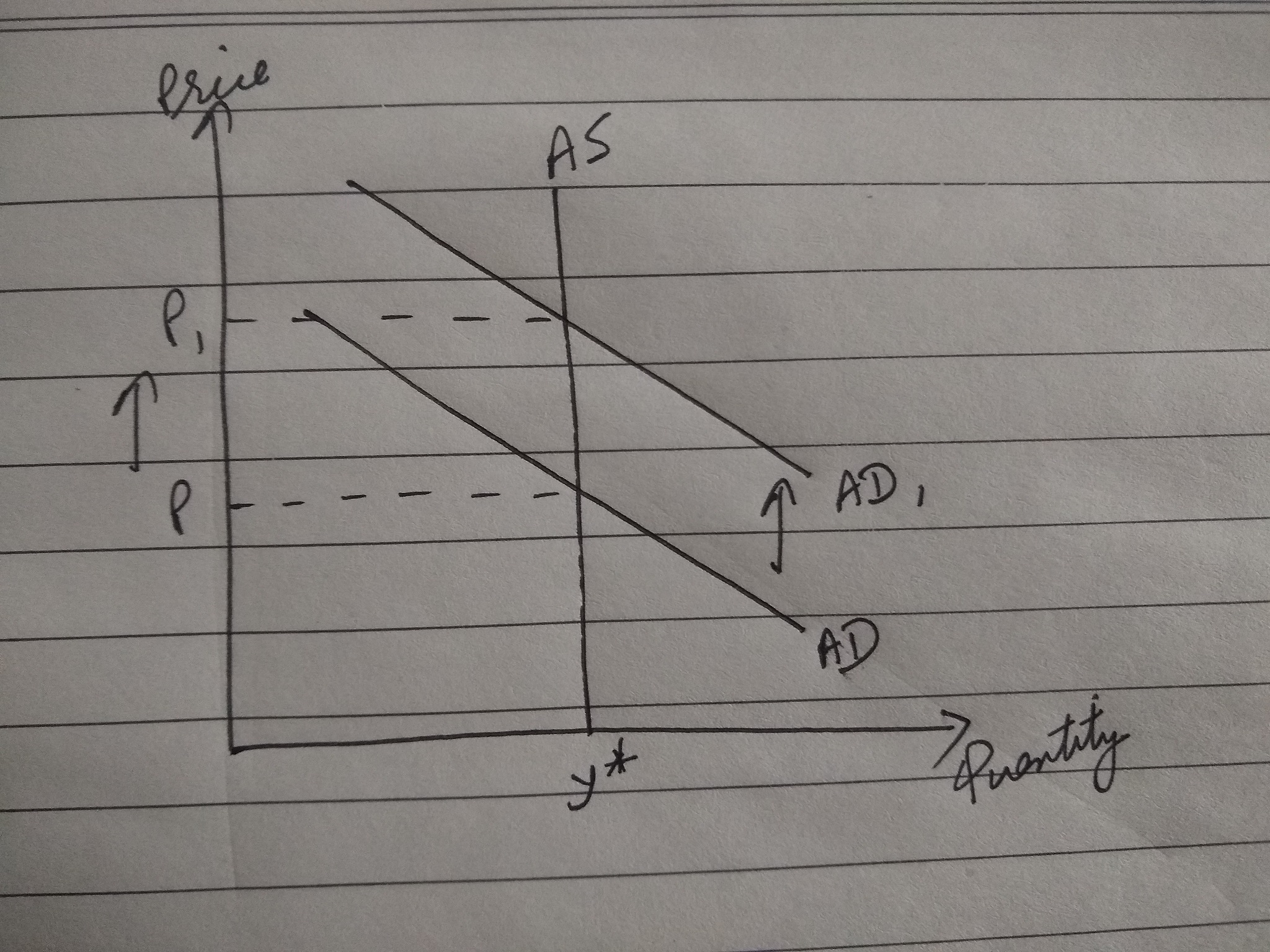#### Earn Coins

Coins can be redeemed for fabulous gifts.

Similar Homework Help Questions
• ### IV. Suppose an economy is in long run equilibrium. (a) Use the model of aggregate demand...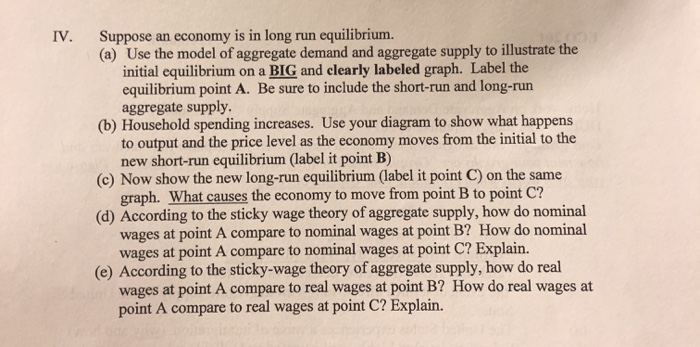IV. Suppose an economy is in long run equilibrium. (a) Use the model of aggregate demand and aggregate supply to illustrate the initial equilibrium on a BIG and clearly labeled graph. Label the equilibrium point A. Be sure to include the short-run and long-run aggregate supply. (b) Household spending increases. Use your diagram to show what happens to output and the price level as the economy moves from the initial to the new short-run equilibrium (label it point B) (c)...

• ### The figure below depicts the aggregate demand curve (AD) and the long-run aggregate supply curve (LRAS) for the United States. The economy is initially at long-run equilibrium, at point A.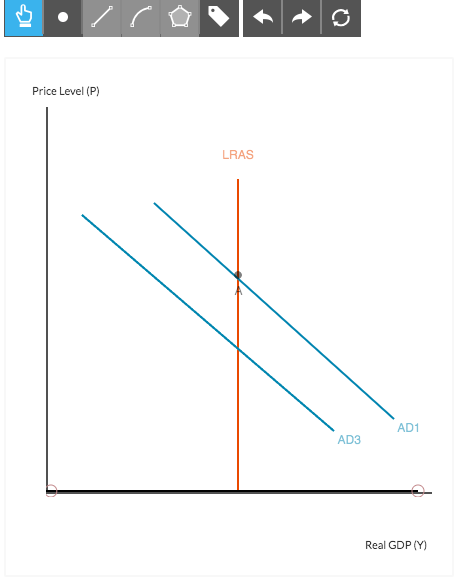The figure below depicts the aggregate demand curve (AD) and the long-run aggregate supply curve (LRAS) for the United States. The economy is initially at long-run equilibrium, at point A.One of the most contentious issues among economists involves the economy’s adjustment to long-run equilibrium. Some economists believe that adjustment can and should occur naturally. This group, the classical economists, stresses the importance of aggregate supply. Others see the return to long-run equilibrium as an adjustment that occurs unpredictably and often...

• ### The figure below depicts the aggregate demand curve (AD), the short-run aggregate supply curve (SRAS), and the long-run aggregate supply curve (LRAS) for the United States. The economy is initially at long-run equilibrium, at point A.The figure below depicts the aggregate demand curve (AD), the short-run aggregate supply curve (SRAS), and the long-run aggregate supply curve (LRAS) for the United States. The economy is initially at long-run equilibrium, at point A.One of the most contentious issues among economists involves the economy’s adjustment to long-run equilibrium. Some economists believe that adjustment can and should occur naturally. This group, the classical economists, stress the importance of aggregate supply. Others see the return to long-run equilibrium as an...

• ### • draw an aggregate demand and aggregate supply diagram to illustrate your answer • show the...

• draw an aggregate demand and aggregate supply diagram to illustrate your answer • show the change in aggregate demand and/or aggregate supply • describe the change(s) you have shown • explain why the adjustments you have described occur. 1. Suppose that there is an expansion of private consumption due to increased optimism about future growth prospects for the economy. (i) Illustrate and explain the effect of this shock in the short-run. (ii) What is the long-run effect likely to...

• ### Using the aggregate demand (AD), the short-run aggregate supply (SRAS), and the long-run aggregate supply (LRAS)...

Using the aggregate demand (AD), the short-run aggregate supply (SRAS), and the long-run aggregate supply (LRAS) curves, briefly explain how an open market purchase will affect the equilibrium price level (P) and real output (Y) in the short run. Assume the economy is initially in a recession?

• ### Using aggregate demand (AD), short-run aggregate supply (SRAS) and long-run aggregate supply (LRAS) curves

Question 1: AD-SRAS-LRAS Model Using aggregate demand (AD), short-run aggregate supply (SRAS) and long-run aggregate supply (LRAS) curves, graphically illustrate the effect of an increase in the money supply on output and prices in the short and long run. Assume that the economy is initially in long run equilibrium at the potential output level and prices are fixed in the short-run. In your graph, label "A" for the initial equilibrium, "B' for the short-run equilibrium, and "C" for the long-run equilibrium.

• ### 11. Using aggregate demand, short-run aggregate sup- ply, and long-run aggregate supply curves, explain the process...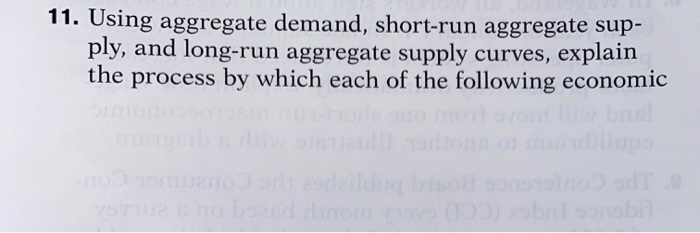11. Using aggregate demand, short-run aggregate sup- ply, and long-run aggregate supply curves, explain the process by which each of the following economic - TEMO alderen events will move the economy from one l. macroeconomic equilibrium to another mu with diagrams. In each case, what are the and long-run effects on the aggregate price lev aggregate output? m one long-run other. Illustrate are the short-run te price level and a. There is a decrease in households' wealth due to decline...

• ### Suppose that the economy is at long-run equilibrium. a. Draw a diagram to illustrate the state of the economy. Be su...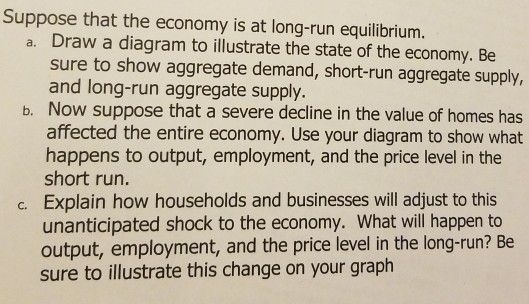Suppose that the economy is at long-run equilibrium. a. Draw a diagram to illustrate the state of the economy. Be sure to show aggregate demand, short-run aggregate supply, and long-run aggregate supply. b. Now suppose that a severe decline in the value of homes has affected the entire economy. Use your diagram to show what happens to output, employment, and the price level in the short run. Explain how households and businesses will adjust to this unanticipated shock to the...

• ### The classical dichotomy and monetary neutrality are represented graphically by  an upward-sloping short-run aggregate-curve.  a vertical long-run aggregate-supply...

The classical dichotomy and monetary neutrality are represented graphically by  an upward-sloping short-run aggregate-curve.  a vertical long-run aggregate-supply curve.  an upward-sloping long-run aggregate-supply curve.  a downward-sloping aggregate-demand curve.

• ### The long-run aggregate supply curve shows that by itself a permanent change in aggregate demand would lead to a long-run...

The long-run aggregate supply curve shows that by itself a permanent change in aggregate demand would lead to a long-run change a. in the price level and output. b. in the price level, but not output. c. in output, but not the price level. d. in neither the price level nor output